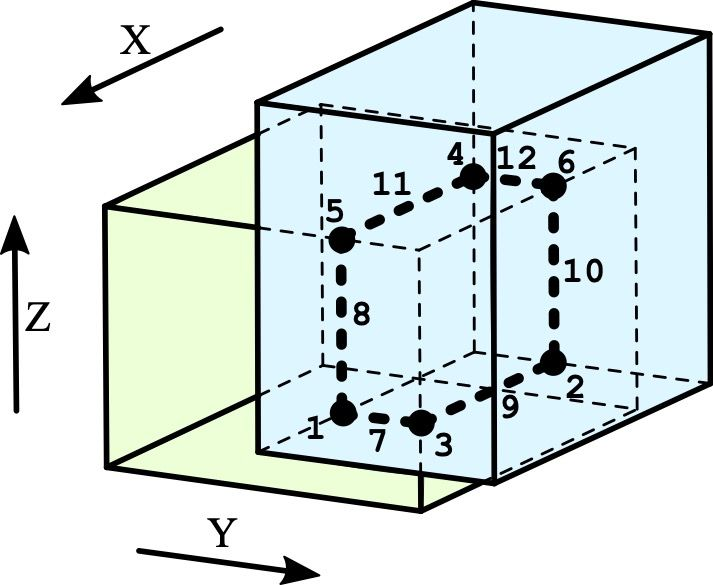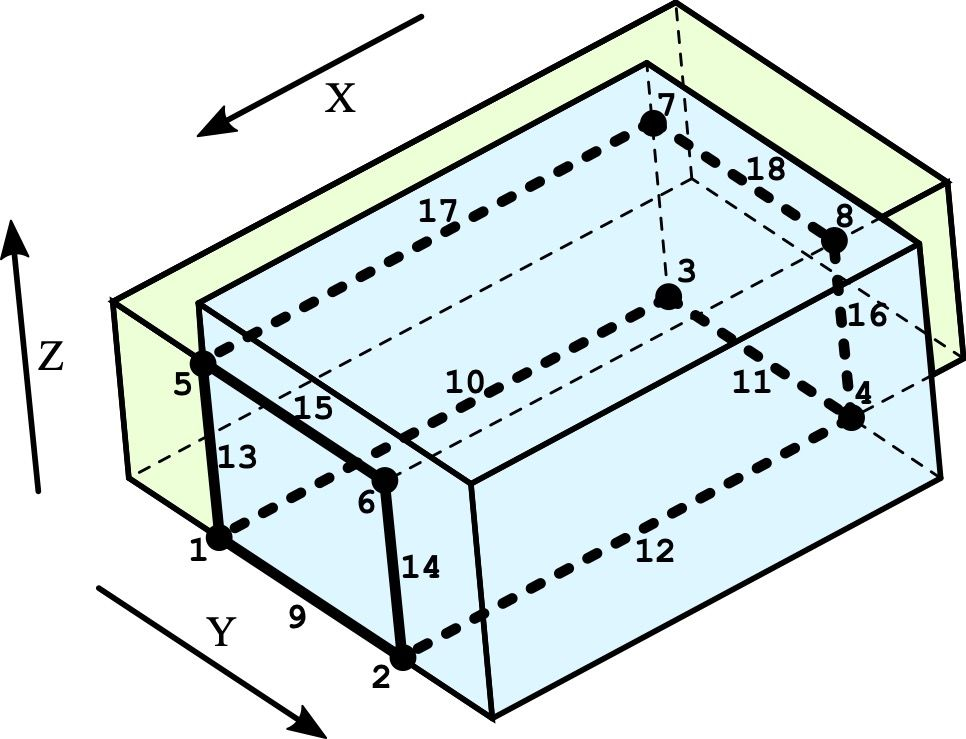시간 제한메모리 제한제출정답맞힌 사람정답 비율
1 초 (추가 시간 없음) 256 MB111100.000%

## 문제

Innokentiy is still very fond of writing geometrical algorithms, but he has switched from triangular meshes to solid bodies. He wants to write an algorithm for building an intersection graph of two bodies, necessary for running, for instance, a boolean operation with these bodies. Note that only the boundaries of the bodies are considered during the building of the graph, and the interior volumes of the bodies are not considered here. Innokentiy knows that building an intersection graph of two arbitrary bodies is a challenging task in the CAD area. He has decided to take it easy: first, he is going to learn to build an intersection graph of two boxes, $A$ and $B$.

A box (usually called axis-aligned box) is a rectangular parallelepiped with sides parallel to the coordinate axes. The boundary of the box consists of topological elements of three types:

• V --- a vertex, which is, geometrically speaking, a point;
• E --- an edge, which is a line segment;
• F --- a face, which is a rectangle;

Each box has 8 vertices, 12 edges, and 6 faces.

For the purpose of this problem, let edges and faces be represented by the corresponding point sets without boundary points. Hence an edge is a line segment which does not contain its end points, and a face is a rectangle which does not contain its corners and sides. Based on this definition, the topological elements have no common points and together form precisely the whole boundary of the box.

An intersection graph consists of interrelated intersection elements defined in the following manner. Consider all possible pairs: a topological element $u$ of the box $A$ and a topological element $v$ of the box $B$ (a total of $676$ pairs). For each such pair ($u$, $v$), find their intersection as intersection of two sets. If this set intersection is non-empty, then it defines the intersection element, which can be of one of the three following types:

• p --- point: a single point;
• c --- curve: a set of points forming a line segment;
• s --- surface: a set of points forming a rectangle;

The intersection graph consists of all intersection elements acquired in this manner.

The intersection elements are interconnected. For each intersection curve, two intersection points located on its ends are saved. For each intersection point, a list of intersection curves incident to that point is saved. An intersection curve is considered incident to its end points and not incident to any other intersection points. For an intersection surface, the set of intersection curves located on its boundary (and thus bounding it) is saved.

## 입력

The first line of the input file contains a single integer $T$ --- the number of test cases ($1 \le T \le 5\,000$). It is followed by $T$ blocks.

Each block consists of two lines: the first line defines the box $A$, while the second line defines the box $B$. A box is described by six integers $x_1$, $y_1$, $z_1$, $x_2$, $y_2$, $z_2$, which do not exceed $10^3$ by absolute value. The numbers $x_1$, $y_1$, $z_1$ define the coordinates of the <<minimal>> vertex, and the numbers $x_2$, $y_2$, $z_2$ define the coordinates of the <<maximal>> vertex ($x_1 < x_2$, $y_1 < y_2$, $z_1 < z_2$).

## 출력

The output file must contain $T$ intersection graphs --- the answers to the test cases from the input file. After each intersection graph, print === (three equality symbols) in a separate line. All numbers in the output file must be integers.

The description of an intersection graph contains a lot of links between intersection elements and topological elements. For the purpose of describing these links, you must assign numbers (or indices) to all elements. All topological elements of the box $A$ must be numbered by integers from $1$ to $26$ in any order. Similarly, all topological elements of the box $B$ must be numbered by integers from $1$ to $26$ in any order. Furthermore, all intersection elements must be numbered from $1$ to $K$ in the order of their appearance in the output file, where $K$ is the total number of intersection elements.

The first line of intersection graph description must contain three numbers: $P$ --- the number of intersection points, $C$ --- the number of intersection curves, and $S$ --- the number of intersection surfaces (naturally, $P + C + S = K$). After that the intersection elements must be described in the following order: first $P$ intersection points, then $C$ intersection curves, and last $S$ intersection surfaces (one intersection element per line).

The description of each intersection element must begin with its index, follower by a three-letter code devised according to the rule:

1. The first letter --- type of intersection element: point p, curve c or surface s.
2. The second letter --- type of topological element $u$: vertex V, edge E or face F.
3. The third letter --- type of topological element $v$.

Here $u$ and $v$ are topological elements from the boxes $A$ and $B$, respectively, from which the intersection element in question was generated. Next, print two integers: the indices of these topological elements $u$ and $v$ in that order. Finally, depending on the type of the intersection element, print additional information.

For an intersection point, additionally provide: three coordinates of its location, the number of incident intersection curves, and the list of indices of these curves. The indices of incident curves may be printed in any order.

For an intersection curve, additionally provide the indices of the two intersection points located on its ends. These two numbers may be printed in any order.

For an intersection surface, additionally provide: the number of intersection curves on its boundary and the list of their indices. The indices of these bounding curves may be printed in any order.

It is not  necessary to separate the numbers in the file strictly by a single space: you can use several spaces in a row, like in the example below.

## 예제 입력 1

2
0 0 0 5 4 3
2 3 1 7 6 5
0 2 6 10 8 9
2 4 6 10 10 10


## 예제 출력 1

6 6 0
1  pFE  14 2  5 3 1  2  7 8
2  pFE  16 4  2 4 1  2  9 10
3  pEF  17 5  5 4 1  2  7 9
4  pFE  22 10  2 3 3  2  11 12
5  pEF  23 11  5 3 3  2  8 11
6  pEF  25 13  2 4 3  2  10 12
7  cFF  14 5   1 3
8  cFF  14 11   1 5
9  cFF  16 5   2 3
10  cFF  16 13   2 6
11  cFF  22 11   4 5
12  cFF  22 13   4 6
===
8 10 2
1  pEV  6 3  10 4 6  3  9 10 13
2  pVE  9 6  10 8 6  3  9 12 14
3  pFV  5 1  2 4 6  2  10 11
4  pEE  8 4  2 8 6  3  11 12 16
5  pEE  23 12  10 4 9  3  13 15 17
6  pVF  26 14  10 8 9  2  14 15
7  pFE  22 10  2 4 9  2  17 18
8  pEF  25 13  2 8 9  2  16 18
9  cEE  6 6   1 2
10  cFE  5 2   1 3
11  cFE  5 4   3 4
12  cEF  8 5   2 4
13  cFE  14 12   1 5
14  cEF  17 14   2 6
15  cEF  23 14   5 6
16  cFF  16 13   4 8
17  cFF  22 11   5 7
18  cFF  22 13   7 8
19  sFF  5 5  4  9 10 11 12
20  sFF  14 14  4  9 13 14 15
===


## 힌트

In the first test case, the boxes poke though each other with a corner. The intersection graph is shown with a bold line. It consists of six points and six curves, obscured to the observer by the box $B$. The indices of the intersection elements are labeled.In the second test case, the boxes share the lower and the front faces, resulting in the intersection surfaces 19 and 20, respectively (they are not shown in the picture). All remaining elements are shown in bold, and their indices are provided.